# [NLP] 相对位置编码(一) Relative Position Representatitons (RPR) - Transformer

1. Self-Attention with Relative Position Representations (Shaw et al.2018): https://arxiv.org/pdf/1803.02155.pdf

2. Attention is all you need (Vaswani et al.2017): https://arxiv.org/pdf/1706.03762.pdf

3. How Self-Attention with Relative Position Representations works: https://medium.com/@_init_/how-self-attention-with-relative-position-representations-works-28173b8c245a

4. [NLP] 相对位置编码(二) Relative Positional Encodings - Transformer-XL: https://www.cnblogs.com/shiyublog/p/11236212.html

## Motivation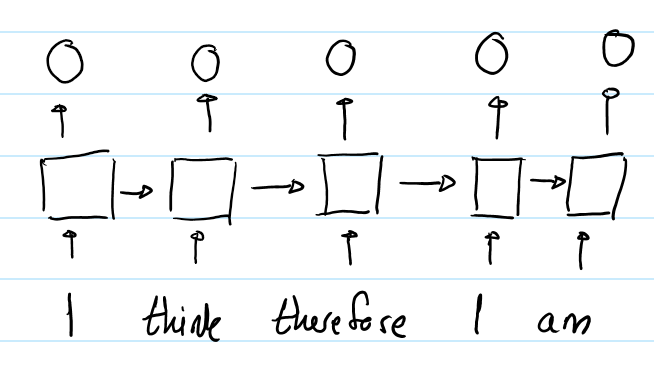RNN中，第一个"I"与第二个"I"的输出表征不同，因为用于生成这两个单词的hidden states是不同的。对于第一个"I"，其hidden state是初始化的状态；对于第二个"I"，其hidden state是编码了"I think therefore"的hidden state。所以RNN的hidden state 保证了在同一个输入序列中，不同位置的同样的单词的output representation是不同的。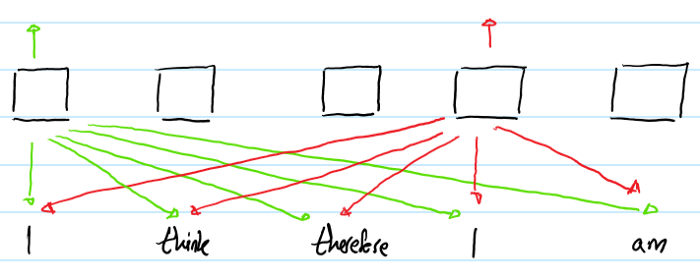## 概述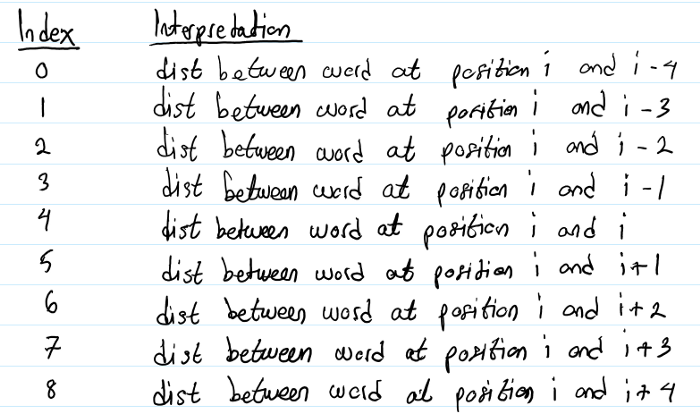1)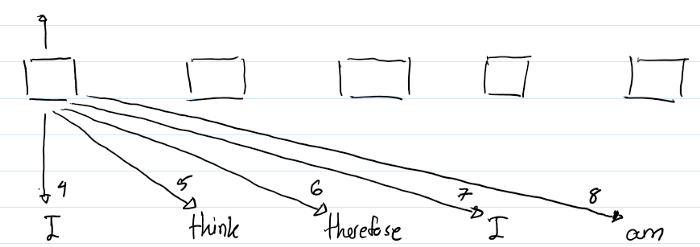2)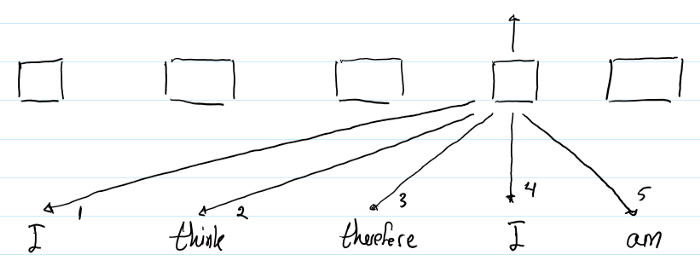## 符号含义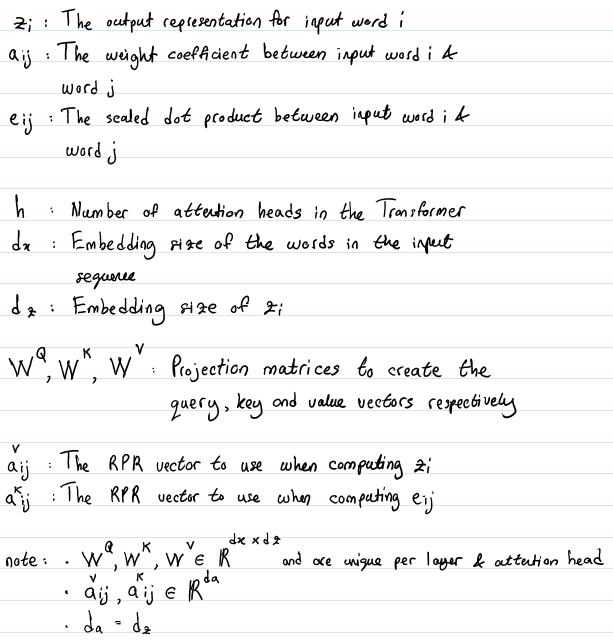1. 有2个RPR的表征。需要在计算$z_i$和$e_{ij}$时分别引入对应的RPR的embedding。计算$z_i$时对应的RPR vector 是$a_{ij}^V$, 计算$e_{ij}时引入的RPR vector$是$a_{ij}^K$. 不同于在做multi-head attention时引入的线性映射矩阵W——对于每个head都不同；这个RPR embedding 在同一层的attention heads之间共享，但是在不同层的RPR可能不同。

2. 最大单词数被clipped在一个绝对的值k以内。向左k个, 再左边均为0， 向右k个，再右边均为k, 所表示的index范围： 2k + 1.

eg. 10 words, k = 3, RPR embedding lookup table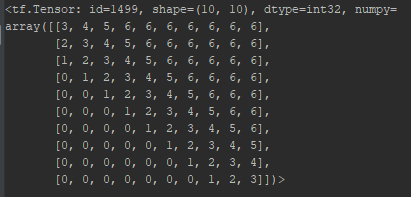1. 作者假设精确的相对位置编码在超出了一定距离之后是没有必要的

2. 截断最大距离使得模型的泛化效果好，可以更好的Generalize到没有在训练阶段出现过的序列长度上。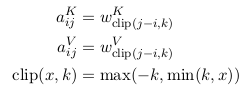$$w^{K} = (w_{-k}^K, ..., w_{k} ^K), w^{V} = (w_{-k}^V, ..., w_{k} ^V)$$

## 实现

1. 若不使用RPR, 计算$z_i$的过程：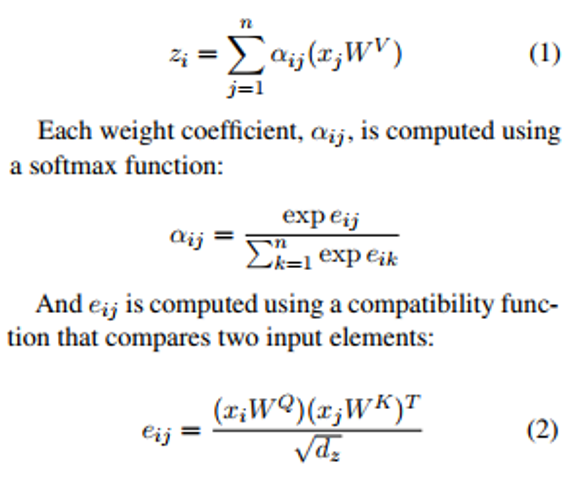2. 若使用RPR，计算$z_i$的过程：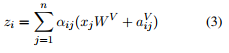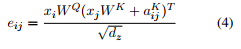(3) 表示在计算word i 的output representation时，对于word j的value vector进行了修改，加上了word i, j 之间的相对位置编码。

(4) 在计算query(i), key(j)的点积时，对key vector进行了修改，加上了word i, j 之间的相对位置编码。

## 高效实现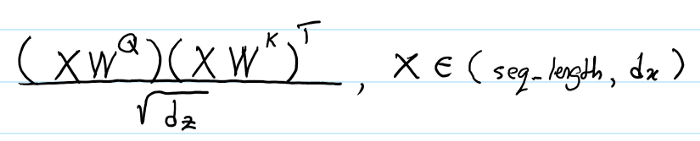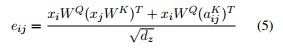(1) 首先看第一项，$$x_iW^Q(x_jW^K)^T$$

(2) A.shape: (seq_length, seq_length, d_a)，

$transpose \rightarrow A^T.shape:$(seq_length, d_a, seq_length)

(3) 第二项中的$x_i W^Q.shape:$ (batch_size, h, seq_length, d_z)

$transpose \rightarrow$ (seq_length, batch_size, h, d_z)

$reshape \rightarrow$ (seq_length, batch_size * h, d_z)

$reshape \rightarrow$(seq_length, batch_size, h, seq_length)

$transpose \rightarrow$ (batch_size, h, seq_length, seq_length)

(3)式的推导同理。

 1 def _relative_attention_inner(x, y, z, transpose):
2   """Relative position-aware dot-product attention inner calculation.
3   This batches matrix multiply calculations to avoid unnecessary broadcasting.
4   Args:
5     x: Tensor with shape [batch_size, heads, length or 1, length or depth].
6     y: Tensor with shape [batch_size, heads, length or 1, depth].
7     z: Tensor with shape [length or 1, length, depth].
8     transpose: Whether to transpose inner matrices of y and z. Should be true if
9         last dimension of x is depth, not length.
10   Returns:
11     A Tensor with shape [batch_size, heads, length, length or depth].
12   """
13   batch_size = tf.shape(x)
15   length = tf.shape(x)
16
17   # xy_matmul is [batch_size, heads, length or 1, length or depth]
18   xy_matmul = tf.matmul(x, y, transpose_b=transpose)
19   # x_t is [length or 1, batch_size, heads, length or depth]
20   x_t = tf.transpose(x, [2, 0, 1, 3])
21   # x_t_r is [length or 1, batch_size * heads, length or depth]
22   x_t_r = tf.reshape(x_t, [length, heads * batch_size, -1])
23   # x_tz_matmul is [length or 1, batch_size * heads, length or depth]
24   x_tz_matmul = tf.matmul(x_t_r, z, transpose_b=transpose)
25   # x_tz_matmul_r is [length or 1, batch_size, heads, length or depth]
26   x_tz_matmul_r = tf.reshape(x_tz_matmul, [length, batch_size, heads, -1])
27   # x_tz_matmul_r_t is [batch_size, heads, length or 1, length or depth]
28   x_tz_matmul_r_t = tf.transpose(x_tz_matmul_r, [1, 2, 0, 3])
29   return xy_matmul + x_tz_matmul_r_t

## 结果[支付宝] 感谢您的捐赠！

But one thing I do: Forgetting what is behind and straining toward what is ahead. ~Bible.Philippians.

posted @ 2019-07-14 20:49  listenviolet  阅读(4442)  评论(0编辑  收藏